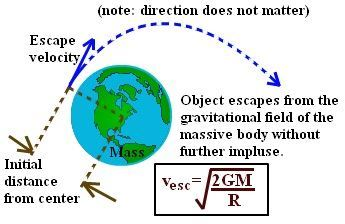# Escape Velocity Formula

## What is Escape Velocity?

Escape velocity is the minimum velocity required by a body to be projected to overcome the gravitational pull of the earth. It is the minimum velocity required by an object to escape the gravitational field that is, escape the land without ever falling back. An object that has this velocity at the earth’s surface will totally escape the earth’s gravitational field ignoring the losses due to the atmosphere.

For example, a spacecraft leaving the surface of Earth needs to go at 7 miles per second, or around 25,000 miles per hour to leave without falling back to the surface.## Formula of Escape Velocity

Escape velocity formula is given

Where,

• V is the escape velocity
• G is the gravitational constant is 6.67408 × 10-11 m3 kg-1 s-2
• M is the mass of the planet
• R is the radius from the center of gravity

An alternative expression for the escape velocity particularly useful at the surface on the body is

Where g is the acceleration due to the gravity of earth.

Hence, Escape velocity is also given by

It is expressed in m/s and the escape velocity of earth is 11,200 m/s.

The escape velocity formula is applied in finding the escape velocity of any body or any planet if mass and radius are known.

### Solved Examples

Example 1

Determine the escape velocity of the Jupiter if its radius is 7149 Km and mass is 1.898 × Kg

Solution:

Given: Mass M = 1.898 × Kg,

Gravitational Constant G = 6.67408 × 10-11 m3 kg-1 s-2

Escape Velocity is given as

Vesc = √2GM / R

=√2 x  6.67408 × 10-11 x  1.898 ×  / 7149

50.3 km/s

Example 2

Determine the escape velocity of the moon if Mass is 7.35 × 1022 Kg and the radius is 1.5 × m.

Solution:

Given

M = 7.35 × 1022 Kg,

R = 1.5 × 106 m

Escape Velocity formula is given by

Vesc = √2GMR

= √2×6.673××7.35×1022 / 1.5×

= 7.59 × m/s